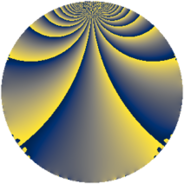Properties

 Label 1575.1.xLevel $1575$ Weight $1$ Character orbit 1575.x Rep. character $\chi_{1575}(451,\cdot)$ Character field $\Q(\zeta_{6})$ Dimension $4$ Newform subspaces $2$ Sturm bound $240$ Trace bound $7$

Related objects

Defining parameters

 Level: $$N$$ $$=$$ $$1575 = 3^{2} \cdot 5^{2} \cdot 7$$ Weight: $$k$$ $$=$$ $$1$$ Character orbit: $$[\chi]$$ $$=$$ 1575.x (of order $$6$$ and degree $$2$$) Character conductor: $$\operatorname{cond}(\chi)$$ $$=$$ $$7$$ Character field: $$\Q(\zeta_{6})$$ Newform subspaces: $$2$$ Sturm bound: $$240$$ Trace bound: $$7$$

Dimensions

The following table gives the dimensions of various subspaces of $$M_{1}(1575, [\chi])$$.

Total New Old
Modular forms 52 10 42
Cusp forms 4 4 0
Eisenstein series 48 6 42

The following table gives the dimensions of subspaces with specified projective image type.

$$D_n$$ $$A_4$$ $$S_4$$ $$A_5$$
Dimension 4 0 0 0

Trace form

 $$4 q + 2 q^{4} + O(q^{10})$$ $$4 q + 2 q^{4} - 2 q^{16} + 6 q^{19} - 2 q^{49} - 6 q^{61} - 4 q^{64} + 2 q^{79} + 6 q^{91} + O(q^{100})$$

Decomposition of $$S_{1}^{\mathrm{new}}(1575, [\chi])$$ into newform subspaces

Label Dim. $$A$$ Field Image CM RM Traces $q$-expansion
$a_{2}$ $a_{3}$ $a_{5}$ $a_{7}$
1575.1.x.a $2$ $0.786$ $$\Q(\sqrt{-3})$$ $D_{6}$ $$\Q(\sqrt{-3})$$ None $$0$$ $$0$$ $$0$$ $$-1$$ $$q+\zeta_{6}q^{4}-\zeta_{6}q^{7}+(\zeta_{6}+\zeta_{6}^{2})q^{13}+\cdots$$
1575.1.x.b $2$ $0.786$ $$\Q(\sqrt{-3})$$ $D_{6}$ $$\Q(\sqrt{-3})$$ None $$0$$ $$0$$ $$0$$ $$1$$ $$q+\zeta_{6}q^{4}+\zeta_{6}q^{7}+(-\zeta_{6}-\zeta_{6}^{2})q^{13}+\cdots$$